# The Catenary

(notes edited by Roberto Bigoni)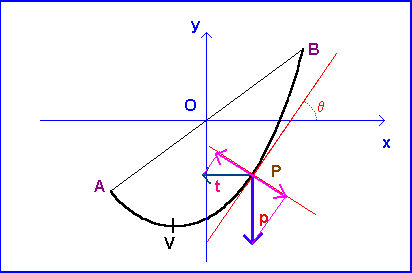A thin, inextensible, perfectly uniform and flexible rope, suspended between the points A and B, in condition of equilibrium in the constant Earth field g, assumes the shape done by a flat curve said Catenary.

In order to deduce analytically the equation of such curve, we can choose a cartesian orthogonal reference system having origin in the middle point of AB, horizontal axis of abscissas and vertical axis of ordinates.

Let P be a point on arc VP. There are two forces wich act on the punctiform rope element in position P, whose length be dl and mass be dm:

• the force t, wich acts leftward, due to the tie A;
• the force p, due to the weight of segment VP, which acts downward.

The condition of equilibrium of the point P is due to the fact that the sum of the tangential components of t and p is balanced by the reaction due to inextensibility of the rope, while the components of p and t perpendicular to the tangent balance themselves: therefore their modules are equal.

If we name θ the angle the geometrical tangent to the point P forms with the axis of the abscissas, we shall have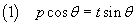Let be y(x) the equation of the curve that describes the disposition of the rope in the chosen system of reference and (x ; y) the cartesian coordinates of the generic P point in such system: tan θ is given by the derivative of the y(x):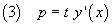The weight p is due to the weight of the segment VP of the rope.

Let v be the abscissa of the vertex, λ the constant linear density of the rope and g the module of the gravitational acceleration: the module of the weight p is given by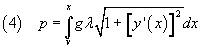and therefore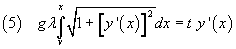Deriving both the terms of (5)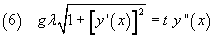and therefore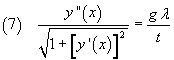If we introduce the constant k: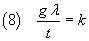the differential equation admits the solution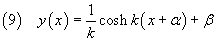The symbol cosh denotes the hyperbolic cosine.

If we know the coordinates a and b of B (and therefore the coordinates of A, symmetrical of B with respect to the origin O) and the length L of the rope (wich obviously must be greater of AB), we have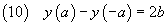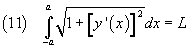From (10) we get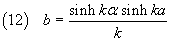and from (11)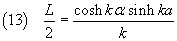Dividing term by term the (12) and (13) we have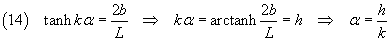and we can express the function (9) in the following form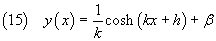From (14) we can also deduce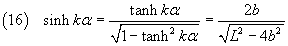Replacing (16) in (12) we obtain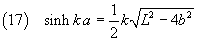Given the values of a, b and L , the equation (17) allows to approximate the value of k (different from the banal solution 0).

Since y(a)=b, from (15) we have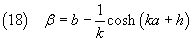Annulling the derivative of y(x) in (15)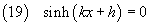we can get the abscissa v of vertex V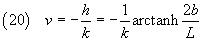The ordinate of V turns out therefore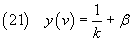The modulus T of the tension, done by the sum of p and t, acting on the point P(x,y) of the rope can be calculated using the Pythagoras's theorem. From (8), (3) e (9) we have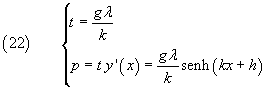therefore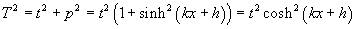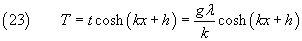Since the value of g is expressed as 9.81 m/s2, the following Javascript application requires measures in metric units.

Parameters of the equation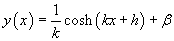input
 abscissa a ordinate b length density
graph
 k h β vertex: x vertex: y
punto
 x ordinate tension

last revision: May 2015